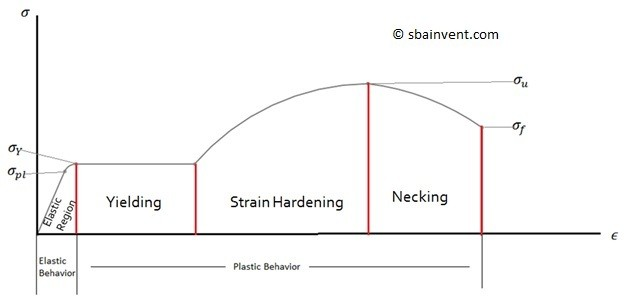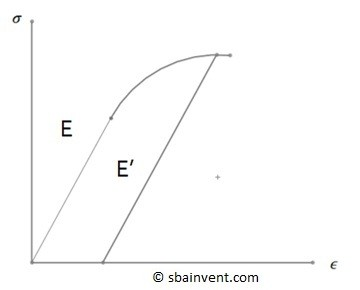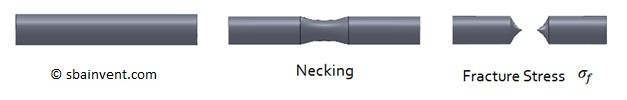# Hook’s Law

Stress and Strain can be related towards one another graphically which can be seen in the stress strain plot below. On the stress strain curve there is an elastic region which exhibits linear behavior, and there is a plastic region which is none linear.##### Elastic Region

The elastic region is the area of the stress strain curve that engineers are most interested in because it exhibits a linear relationship which is known as Hooke’s Law, equation 1. Due to this linear relationship a constant known as the Young’s Modulus can be derived which can than be used mathematically to relate stress and strain to one another.

(Eq 1)  $σ=Eε$

σ = stress

ε = strain

E = Young’s Modulus

##### Plastic Region

The plastic region is the region of the stress strain curve where Hook’s law will no longer apply due to the fact that region is non linear. The plastic region consists of three different parts, which are Yielding, Strain Hardening, and Necking. Refer to the image below.In the Yielding section the part under load will start to deform. However, the load will stay the same, which means the stress on the part will be the same even though the amount the part is deflecting is increasing due to strain. The next section, Strain Hardening, additional loading will occur on the object, however stress and strain will no long be linearly related. During strain hardening, if the part is unloaded, the Young’s Modulus (Elastic Region) will shift. Due to this shift a larger force on the object will be required to cause the part to continue to deform. However, this also brings the ultimate stress closer to the point where the part will start to yield.Finally, necking will occur after the ultimate stress has been reached. Necking will continue until the part reaches its fracture stress and breaks.The above covered the basics of Hook’s law. In this section you can also learn how energy is relates to Hook’s law, how Poisson’s Ratio is used to relate Young’s Modulus to the Shear Modulus, and finally, how the stress-strain curve can be used to determine if a material isductile or brittle.